﻿ 基于Otsu和FMM方法的风洞试验图像修复Download PDF文章快速检索 高级检索

Image inpainting of wind tunnel test based on Otsu method and FMM
JIANG Tao, LI Qiang, CHEN Suyu, CHANG Yu, ZHANG KouliHypervelocity Aerodynamics Institute, China Aerodynamics Research and Development Center, Mianyang 621000, China
Abstract: Many kinds of optics test technologies have been applied broadly in wind tunnel test, but the breakages and irrelevant information of images could affect data processing and analysis. Taking schlierern images with defects as an example, the image inpainting technology is studied. According to the characteristics of the image defects such as discrete, variable in shape and size, low gray levels of core regions and large gray gradients on the edges of defects, the image inpainting workflow is divided into pending regions determination, defects identification, and defects inpainting. The Otsu method is used to segment images in Step 1 and Step 2, and the Fast Marching Method (FMM) is applied to inpaint the defects in Step 3. After image inpainting, the defects of schlieren images are automatically identified and reduced or weakened, and the inpainting information is objective and reasonable. This image inpainting method could be extended to other optics test technologies in wind tunnel test.
Keywords: image inpainting    Otsu method    fast marching method    schlieren    optics    wind tunnel test

1 图像缺陷的特征及修复流程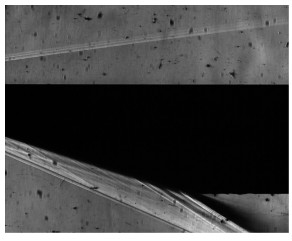图 1 纹影图像 Fig. 1 Schlieren image

2 算法 2.1 图像分割算法

 ${\omega _0} = \omega (k) = \sum\limits_{i = 1}^k {{p_i}}$ （1）

 ${\mu _0} = \sum\limits_{i = 1}^k i {p_i}/{\omega _0}$ （2）

 ${\omega _1} = \sum\limits_{i = k + 1}^L {{p_i}}$ （3）

 ${\mu _1} = \sum\limits_{i = k + 1}^L i {p_i}/{\omega _1}$ （4）

 ${\mu _{\rm{T}}} = \mu (L) = \sum\limits_{i = 1}^L i {p_i} = {\omega _0}{\mu _0} + {\omega _1}{\mu _1}$ （5）

 $\begin{array}{l} \sigma _{\rm{B}}^2 = {\omega _0}{\left( {{\mu _0} - {\mu _{\rm{T}}}} \right)^2} + {\omega _1}{\left( {{\mu _1} - {\mu _{\rm{T}}}} \right)^2} = \\ \;\;\;\;\;\;{\omega _0}{\omega _1}{\left( {{\mu _1} - {\mu _0}} \right)^2} \end{array}$ （6）

2.2 图像修复算法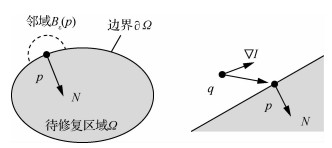图 2 修复原理 Fig. 2 Inpainting principle
 $I(p) = \frac{{\sum\nolimits_{q \in {B_\varepsilon }(p)} w (p,q)[I(q) + \nabla I(q)(p - q)]}}{{\sum\nolimits_{q \in {B_\varepsilon }(p)} w (p,q)}}$ （7）

 $w(p,q) = {\rm dir}(p,q) \cdot {\rm dir}(p,q) \cdot {\rm lev}(p,q)$ （8）

 ${\rm dir}(p,q) = \frac{{p - q}}{{\left\| {p - q} \right\|}} \cdot N(p)$
 ${\rm dst}(p,q) = \frac{{d_0^2}}{{{{\left\| {p - q} \right\|}^2}}}$
 ${\rm lev}(p,q) = \frac{{{T_0}}}{{1 + \left| {T(p) - T(q)} \right|}}$

1) Boundary。待修复区域边界∂Ω上的点，T = 0，其T值将被更新。

2) Known。∂Ω外已知区域的像素，其T值和灰度值I已知。

3) Inside。∂Ω内部的像素，其T值和灰度值I未知。

FMM就是对待修复区域内的点解Eikonal方程：

 $\left| {\nabla T} \right| = 1$ （9）

D±xD±y分别为x方向和y方向的差分，方程的稳定解为

 $\begin{array}{l} \max {\left( {{D^{ - x}}T, - {D^{ + x}}T,0} \right)^2} + \\ \;\;\;\;\;\;\max {\left( {{D^{ - y}}T, - {D^{ + y}}T,0} \right)^2} = 1 \end{array}$ （10）

 $\left\{ {\begin{array}{*{20}{l}} {{D^{ - x}}T(i,j) = T(i,j) - T(i - 1,j)}\\ {{D^{ + x}}T(i,j) = T(i + 1,j) - T(i,j)}\\ {{D^{ - y}}T(i,j) = T(i,j) - T(i - 1,j)}\\ {{D^{ + y}}T(i,j) = T(i + 1,j) - T(i,j)} \end{array}} \right.$

3 图像修复试验

3.1 图像分割试验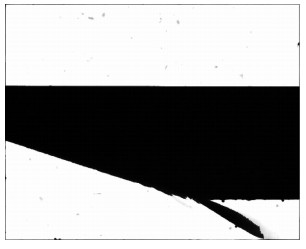图 3 待处理区域确定 Fig. 3 Determination of pending regions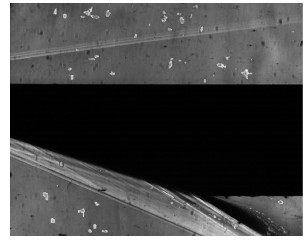图 4 图像缺陷识别(部分结果) Fig. 4 Partial result of image defects identification
3.2 图像修复试验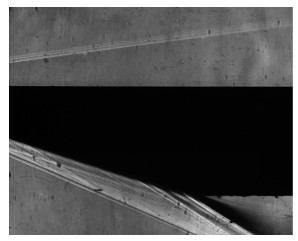图 5 图像修复结果 Fig. 5 Image inpainting result
4 评估与验证 4.1 修复前后对比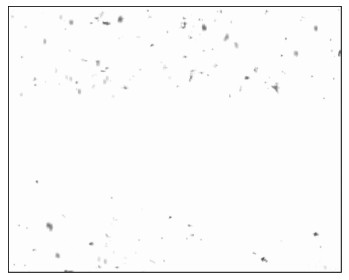图 6 图像修复前后对比 Fig. 6 Comparison of images before and after inpainting
4.2 应用验证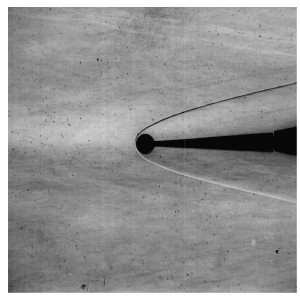图 7 有缺陷的纹影图像 Fig. 7 Schlieren image with defects图 8 图 7缺陷识别结果 Fig. 8 Image defects identification result of Fig. 7图 9 图 7修复结果 Fig. 9 Image inpainting result of Fig. 7图 10 图 7中存在的缺陷 Fig. 10 Image defects of Fig. 7
4.3 定量评估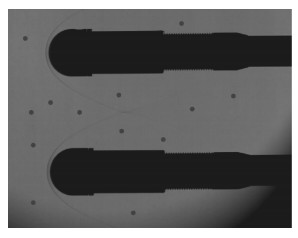图 11 添加缺陷的图像 Fig. 11 Image with artificial defects

 像表类型 像素量 灰度值 缺陷像素 1 040 65 修复像素 1 634 71~117108.24(均值) 原始像素 1 634 70~119107.45(均值) 修复像素和原始像素的灰度值差异 1~111.95(均值)

 灰度差异 像素量 比例/% 1 744 45.53 2 502 30.72 3 238 14.57 4~6 139 8.51 7~11 11 0.67
5 结论

1) 经数字图像修复技术处理，纹影图像的缺陷明显减少或得到削弱，可将缺陷图像修复至与原始图像相当的水平。

2) 图像修复的信息是客观合理的，没有破坏或改变模型形状和流场结构等关键信息，对非缺陷区域和图像整体的影响极小。

3) 在风洞试验中引入数字图像修复是可行的，图像修复方法具有通用性，也可根据不同图像的特点选用不同的算法，推广运用于其他光学测试技术，如修复TSP和PSP技术中的标记点、涂层脱落或污染产生的暗区等。

  杨祖清. 流动显示技术[M]. 北京: 国防工业出版社, 2002: 93-276. YANG Z Q. Flow visualization[M]. Beijing: National Defense Industry Press, 2002: 93-276. (in Chinese)  李桂春. 气动光学[M]. 北京: 国防工业出版社, 2002: 347-489. LI G C. Aero-optics[M]. Beijing: National Defense Industry Press, 2002: 347-489. (in Chinese)  李强, 江涛, 陈苏宇, 等. 激波风洞边界层转捩测量技术及应用[J]. 航空学报, 2019, 40(8): 122740. LI Q, JIANG T, CHEN S Y, et al. Measurement technology and measuring of boundary layer transition in shock tunnel[J]. Acta Aeronautica et Astronautica Sinica, 2019, 40(8): 122740. (in Chinese) Cited By in Cnki | Click to display the text  战培国, 曾慧, 钟萍, 等. 2017年国外风洞试验发展动态综述[J]. 飞航导弹, 2018(8): 16-48. ZHAN P G, ZENG H, ZHONG P, et al. Review of the development of foreign wind tunnel tests in 2017[J]. Aerodynamic Missile Journal, 2018(8): 16-48. (in Chinese) Cited By in Cnki | Click to display the text  GABRIELE B, GUILLAUME G, TAMAS R, et al. Optical characterization of boundary layer transition[C]//17th International Symposium on Applications of Laser Techniques to Fluid Mechanics, 2014.  张扣立, 周嘉穗, 孔荣宗, 等. CARDC激波风洞TSP技术研究进展[J]. 空气动力学学报, 2016, 34(6): 738-743. ZHANG K L, ZHOU J S, KONG R Z, et al. Development of TSP technique in shock tunnel of CARDC[J]. Acta Aerodynamica Sinica, 2016, 34(6): 738-743. (in Chinese) Cited By in Cnki (1) | Click to display the text  韩曙光, 贾广森, 文帅, 等. 磷光热图技术在常规高超声速风洞热环境实验中的应用[J]. 气体物理, 2017, 2(4): 56-63. HAN S G, JIA G S, WEN S, et al. Heat transfer measurement using a quantitative phosphor thermography system in blowdown hypersonic facility[J]. Physics of Gases, 2017, 2(4): 56-63. (in Chinese) Cited By in Cnki | Click to display the text  刘祥.双组份压敏漆试验技术测量不确定度研究[D].绵阳: 中国空气动力研究与发展中心, 2016. LIU X. Investigation of pressure uncertainty for two-component pressure sensitive paint[D]. Mianyang: China Aerodynamics Research and Development Center, 2016(in Chinese). Cited By in Cnki | Click to display the text  BERTALMIO M, SAPIRO G, CASELLES V, et al. Image inpainting[C]//Proceedings SIGGRAPH 2000, Computer Graphics Proceedings, Annual Conference Series, 2000: 417-424.  GUILLEMOT C, LE MEUR O. Image inpainting:Overview and recent advances[J]. IEEE Signal Processing Magazine, 2014, 31(1): 127-144. Click to display the text  张红英.数字图像修复技术的研究与应用[D].成都: 电子科技大学, 2006: 1-32. ZHANG H Y. Research and application digital image inpainting[D]. Chengdu: University of Electronic and Technology of China, 2006: 1-32(in Chinese). Cited By in Cnki (1) | Click to display the text  张丽莹.数字图像修复算法研究[D].天津: 天津大学, 2015: 1-18. ZHANG L Y. The study of digital image completion algorithms[D]. Tianjin: Tianjin University, 2015: 1-18(in Chinese).  SHIH T K, CHANG R C, LU L C, et al. Multi-layer inpainting on Chinese artwork restoration applications[C]//IEEE International Conference on Multimedia and Expo. Piscataway, NJ: IEEE Press, 2004.  焦莉娟, 王文剑, 李秉婧, 等. 改进的块匹配五台山壁画修复算法[J]. 计算机辅助设计与图形学学报, 2019, 31(1): 118-125. JIAO L J, WANG W J, LI B J, et al. Wutai Mountain mural inpainting based on improved block matching algorithm[J]. Journal of Computer-Aided Design & Computer Graphics, 2019, 31(1): 118-125. (in Chinese) Cited By in Cnki | Click to display the text  罗希平, 田捷, 诸葛婴, 等. 图像分割方法综述[J]. 模式识别与人工智能, 1999, 12(3): 300-312. LUO X P, TIAN J, ZHUGE Y, et al. A survey on image segmentation techniques[J]. Pattern Recognition and Artificial Intelligence, 1999, 12(3): 300-312. (in Chinese) Cited By in Cnki | Click to display the text  林开颜, 吴军辉, 徐立鸿. 彩色图像分割方法综述[J]. 中国图象图形学报, 2005, 10(1): 1-10. LIN K Y, WU J H, XU L H. A survey on color image segmentation techniques[J]. Journal of Image and Graphics, 2005, 10(1): 1-10. (in Chinese) Cited By in Cnki (758) | Click to display the text  韩思奇, 王蕾. 图像分割的阈值法综述[J]. 系统工程与电子技术, 2002, 24(6): 91-94. HAN S Q, WANG L. A survey of thresholding methods for image segmentation[J]. Systems Engineering and Electronics, 2002, 24(6): 91-94. (in Chinese) Cited By in Cnki (1110) | Click to display the text  SEZGIN M, SANKUR B. Survey over image thresholding techniques and quantitative performance evaluation[J]. Journal of Electronic Imaging, 2004, 13(1): 146-165. Click to display the text  NOBUYUKI O. A threshold selection method from gray-level histogram[J]. IEEE Transactions on Systems, Man and Cybernetics, 1979, 9(1): 62-66. Click to display the text  付忠良. 图像阈值选取方法——Otsu方法的推广[J]. 计算机应用, 2000, 20(5): 37-39. FU Z L. Methods of selecting image threshold-Extension of Otsu method[J]. Computer Applications, 2000, 20(5): 37-39. (in Chinese) Cited By in Cnki (581) | Click to display the text  刘艳, 赵英良. Otsu多阈值快速求解算法[J]. 计算机应用, 2011, 31(12): 3363-3365. LIU Y, ZHAO Y L. Quick approach of multi-threshold Otsu method for image segmentation[J]. Journal of Computer Applications, 2011, 31(12): 3363-3365. (in Chinese) Cited By in Cnki (32) | Click to display the text  申铉京, 刘翔, 陈海鹏. 基于多阈值Otsu准则的阈值分割快速计算[J]. 电子与信息学报, 2017, 39(1): 144-149. SHEN X J, LIU X, CHEN H P. Fast computation of threshold based on multi-threshold Otsu criterion[J]. Journal of Electronics & Information Technology, 2017, 39(1): 144-149. (in Chinese) Cited By in Cnki (4) | Click to display the text  周迪, 夏哲雷. 一种改进的Otsu阈值分割算法[J]. 中国计量大学学报, 2016, 23(1): 319-323. ZHOU D, XIA Z L. An improved Otsu threshold segmentation algorithm[J]. Journal of China University of Metrology, 2016, 23(1): 319-323. (in Chinese) Cited By in Cnki (65) | Click to display the text  李开宇, 孙玉刚. 引入连续性强度和置信度因子的快速图像修复[J]. 中国图象图形学报, 2012, 17(4): 465-470. LI K Y, SUN Y G. Fast image inpainting algorithm introducing continuous strength and confidence factor[J]. Journal of Image and Graphics, 2012, 17(4): 465-470. (in Chinese) Cited By in Cnki (9) | Click to display the text  闵溪青, 黄杰. 简化的快速图像修复方法[J]. 计算机应用, 2017, 37(S1): 169-172. MIN X Q, HUANG J. Simplified fast image inpainting method[J]. Journal of Computer Applications, 2017, 37(S1): 169-172. (in Chinese) Cited By in Cnki | Click to display the text  SETHIAN J A. A fast marching level set method for monotonically advancing fronts[J]. National Academy of Sciences, 1996, 93(4): 1591-1595. Click to display the text  TELEA A. An image technique based on the fast matching method[J]. Journals of Graphics Tools, 2004, 9(1): 23-34. Click to display the text
http://dx.doi.org/10.7527/S1000-6893.2019.23293

0

#### 文章信息

JIANG Tao, LI Qiang, CHEN Suyu, CHANG Yu, ZHANG Kouli

Image inpainting of wind tunnel test based on Otsu method and FMM

Acta Aeronautica et Astronautica Sinica, 2020, 41(2): 123293.
http://dx.doi.org/10.7527/S1000-6893.2019.23293